• 输出层误差计算公式 III . 隐藏层层误差计算公式 IV . 使用误差更新 连接权值 V . 使用误差更新 单元偏置 VI . 反向传播 过程 VII . 损失函数 简介 VIII . 损失函数 IX . 损失函数 举例 X . 损失函数 优化过程
文章目录I . 向后传播误差 简介II . 输出层误差计算公式III . 隐藏层层误差计算公式IV . 使用误差更新 连接权值V . 使用误差更新 单元偏置VI . 反向传播 过程VII . 损失函数 简介VIII . 损失函数IX . 损失函数 举例X . 损失函数 优化过程

I . 向后传播误差 简介

1 . 后向传播误差 : 计算每层每个单元的误差 , 根据该误差更新 权值 和 偏置 设置 ;

2 . 计算误差的目的 : 使用计算出来的误差 , 更新单元连接的 权值 , 和 单元 本身的偏置 参数 , 用于实时反映出前向传播输入的误差 ;

II . 输出层误差计算公式

输出层误差计算 :

① 输出层单元 $j$ , 误差计算公式 :
$Err_j = O_j ( 1 - O_j ) ( T_j - O_j )$

$O_j$ 是单元 $j$ 的输出 ;

$T_j$ 是样本分类的真实的属性值 , 取值 0 或 1 , 输出层每个单元的节点输出都是 0 或 1 , 如果分类有多个离散值 , 那么输出层使用多个节点表示这些分类 ;

② 公式来源 : 该公式来源于 损失函数 , 对损失函数进行求导 ;

III . 隐藏层层误差计算公式

隐藏层误差计算 :

$Err_j = O_j ( 1 - O_j ) ( \sum_{k=1}^n Err_k w_{jk} )$

$O_j$ 是本层单元 $j$ 的输出 ;

$Err_k$ 是下一层第 $k$ 个单元的误差 ;

$w_{jk}$ 是本单元 与 下一层第 $k$ 个单元连接的 权值 ;

$\sum_{k=1}^n Err_k w_{jk}$ 是一个线性组合 , 本层的 $j$ 单元 , 连接下一层的 $n$ 个单元 , 计算下层每个节点的误差 $Err_k$ , 乘以连接的权值 $w_{jk}$ , 再将多个下层节点的 $Err_k w_{jk}$ 计算值累加 ;

IV . 使用误差更新 连接权值

1 . 计算误差的目的 : 使用计算出来的误差 , 更新单元连接的 权值 , 和 单元 本身的偏置 参数 , 用于实时反映出前向传播输入的误差 ;

2 . 权值更新公式 : 修改 单元 $i$ 和 单元 $j$ 连接的权值 , 注意连接方向是 单元 $i$ 连接到单元 $j$ , $i$ 是 $j$ 的前一层 ;

$\Delta w_{ij} = (l) Err_j O_i$
$w_{ij}' = w_{ij} + \Delta w_{ij}$

$\Delta w_{ij}$ 是 单元 $i$ 和 单元 $j$ 的连接的权值的改变值 ;
$l$ 是学习率 , 一般是 $0.8$ , $0.9$ 等 $(0,1)$ 区间内的数值 ;
$Err_j$ 是单元 $j$ 的误差 ;
$O_i$ 表示 单元 $i$ 的输出 ;
$Err_j O_i$ 是通过求导得出的梯度 ;
$w_{ij}'$ 表示新的权值 ;
$w_{ij}$ 表示老的权值 ;

3 . 连接权值更新总结 : 该公式是梯度公式 , 后向传播算法是梯度下降算法 , 其权值更新是 旧的权值 , 加上权值的改变 , 计算出新的连接权值 ;

V . 使用误差更新 单元偏置

1 . 计算误差的目的 : 使用计算出来的误差 , 更新单元连接的 权值 , 和 单元 本身的偏置 参数 , 用于实时反映出前向传播输入的误差 ;

2 . 偏置更新公式 : 修改 单元 $j$ 的偏置 ;
$\Delta \theta_j = (l) Err_j$
$\theta' = \theta_j + \Delta \theta_j$

$\Delta \theta_j$ 是偏置的改变 ;
$l$ 是学习率 , 一般是 $0.8$ , $0.9$ 等 $(0,1)$ 区间内的数值 ;
$Err_j$ 是单元 $j$ 的误差 ;
$\theta'$ 是新的偏置 ;
$\theta$ 是老的偏置 ;

3 . 偏置更新总结 : 当前节点的误差 , 乘以学习率 , 就是偏置的改变 ; 旧的偏置值 , 加上偏置改变 , 可计算出新的偏置值 ;

VI . 反向传播 过程

1 . 权值 偏置 更新操作 : 先计算误差 , 然后根据误差计算 权值 和 偏置的改变值 , 再将原来的 权值 和 偏置 值 加上对应的改变值 , 计算出新的权值和偏置值 ;

2 . 反向传播的过程 : 将误差从后向前传播 , 根据误差 , 从后到前依次修改权值和偏置值 ;

① 向后传播误差本质 : 使用梯度下降方法 , 优化损失函数 , 使损失函数取最小值 , 在这个过程中 , 不停地迭代修改 单元连接权值 , 和 每个单元的偏置 ;
② $3$ 种梯度下降方法 :  随机梯度下降 , 批量梯度下降 , 小批量梯度下降方法 ;
③ 损失函数 : 类似于评分函数 ;  如 误差平方和 ;
④ 两个核心 : 首先 , 采用什么样的损失函数 , 其次 , 如何进行迭代修改 权值和偏置 ;

VII . 损失函数 简介

1 . 损失函数 作用 :

① 训练输出 : 神经网络 学习训练样本有一个输出输出 ;
② 样本实际值对应输出 : 数据集样本的真正的属性值对应的输出 , $0$ 或 $1$ ;
③ 引入损失函数 : 使用损失函数 计算 上述 训练输出 和 样本实际值对应输出 的差别 ;
④ 损失函数最小值 : 训练输出 和 样本实际值对应输出 的差别越小越好 , 因此损失函数进行优化时 , 损失函数的值越小越好 ;

2 . 损失函数优化 :

① 损失函数 优化过程 : 在优化使损失函数取最小值的过程 , 就是使对应的 单元连接权值 , 和 单元的偏置 , 等参数不断优化的过程 ;
② 损失函数最小值 与 最佳参数 : 最终损失函数最小值的状态的 权值 和 偏置就是 学习出的最佳参数值 ;

3 . 损失函数本质 : 损失函数 最小值 计算过程 , 就是通过 梯度下降方法 , 逐步迭代获取 最佳 权值 与 偏置过程 ;

VIII . 损失函数

1 . 损失函数作用 : 度量 预测结果 与 实际结果 差异 ;
① 神经网络学习训练目的 : 使 损失函数 取值最小 ;
② 损失函数要求 : 预测结果越好 , 损失越小 ;

2 . 损失函数选择 :

① 分布比较 : 比较的两个属性是 分布 , 那么使用 交叉熵 损失函数 ;
② 数值比较 : 如果是两个 数值属性 之间比较 , 使用 误差平方和 损失函数 ;

IX . 损失函数 举例

1 . 样本示例 :

① 样本个数 : $n$ 个 ;
② 样本属性 : 取值有两种 , $0$ 或 $1$ , 即样本的属性值只能从 $\{0, 1\}$ 集合中取值 ;
③ 实际属性值 : $y_i$ 为实际属性值 , 并且有 $y_i \in \{0, 1\}$ ;
④ 预测属性值 : $x_i$ 为预测属性值 , 并且有 $x_i \in [0, 1]$ , 在 误差平方和 ( Mean squared error ) 损失函数中 , $x_i$ 取值范围可以是全体实数 ;

2 . 误差平方和 ( 均方误差 Mean Squared Error ) 损失函数

误差平方和公式 : 误差平方和 , 又叫均方误差 , 英文全称 Mean squared error , 简称 MSE ;

$误差平方和 = \dfrac{1}{n} \sum_{i = 1}^{n} (x_i - y_i)^2$

预测属性 减去 实际属性 得到差值 , 将该差值平方 , 目的是去掉差值的符号 ( 正负号 ) , 得到误差平方 , 再将 $n$ 个误差平方加起来 , 得到平方和 , 然后除以 $n$ 取平均值 , 即得到 $n$ 个样本的 平均的 误差平方 , 因此叫做 均方误差 , 又叫误差平方和 ;

3 . 交叉熵 ( Cross Entropy ) 损失函数

交叉熵公式 :

$交叉熵 = - \dfrac{1}{n} \sum_{i = 1}^{n} [ y_i \times log(x_i) + (1 - y_i) \times log(1 - x_i) ]$

该 交叉熵公式 通常用于比较分布之间的差别 ;

X . 损失函数 优化过程

1 . 损失函数作用 : 损失函数的目的是为神经网络优化 每个连接的 权值 和 每个单元的 偏置 , 使数据集的损失函数最小 ;

2 . 损失函数优化注意事项 :
① 参数个数 : 参数数量很多 , 搜索算法空间很大 , 可能有百万级 ;
② 参数取值 : 参数取值范围很广 , 取值范围从 负无穷 到 正无穷 ;
③ 损失函数复杂 : 损失函数 与 参数 的关系很复杂 ;
④ 计算能力 : 对于海量的大数据 , 训练时不能一次性训练所有的数据 , 计算能力也是有限制的 ;
⑤ 过拟合问题  : 训练集上损失函数达到最小值 , 在测试模型时 , 不一定能得到该结果 ;


展开全文数据挖掘 神经网络 后向传播算法 损失函数 反向传播
• 一个潜在疑问是：如何判断应该使用均方误差还是总平方误差（或绝对误差）？ 总平方误差是指每个点的误差之和，方程式为： M=∑i=1m12(y−y^)2M = \sum_{i=1}^m\frac{1}{2}(y-\hat{y})^2M=∑i=1m​21​(y−y^​)2 ...
一个潜在疑问是：如何判断应该使用均方误差还是总平方误差（或绝对误差）？
总平方误差是指每个点的误差之和，方程式为：
$M = \sum_{i=1}^m\frac{1}{2}(y-\hat{y})^2$
均方误差是指这些误差的平均值，方程式为：
$T = \sum_{i=1}^m\frac{1}{2m}(y-\hat{y})^2$
其中 m是数据点的数量，
好消息是，选择哪个并不重要。可以看出，总平方误差是多个均方误差相加的结果，因为
$M=mT$
因此，既然导数是线性方程， T的梯度也是 m乘以 M的梯度。
但是，梯度下降步骤包括减去误差的梯度乘以学习速率$\alpha$。因此，选择均方误差还是总平方误差只是选择不同的学习速率。
在现实中，我们可以借助算法判断什么样的学习速率比较合适。因此，如果我们使用均方误差或总平方误差，算法将只是选择不同的学习速率。


展开全文均方误差
• ## 平方误差之和SSE

万次阅读 2018-07-26 00:05:42
下面这张图是一个简单的线性回归模型,图中标注了每个样本点预测值与真实值的残差。...解析：SSE 是平方误差之和（Sum of Squared Error），SSE = (-0.2)^2 + (0.4)^2 + (-0.8)^2 + (1.3)^2 + (-0.7)^2 = 3.02...
下面这张图是一个简单的线性回归模型,图中标注了每个样本点预测值与真实值的残差。计算 SSE 为多少？A. 3.02

B. 0.75

C. 1.01

D. 0.604

答案：A

解析：SSE 是平方误差之和（Sum of Squared Error），SSE = (-0.2)^2 + (0.4)^2 + (-0.8)^2 + (1.3)^2 + (-0.7)^2 = 3.02
展开全文SSE
• 最小平方误差判别准则函数 对于上一节提出的不等式组： 在线性不可分的情况下，不等式组不可能同时满足。一种直观的想法就是，希望求一个a*使被错分的样本尽可能少。这种方法通过求解线性不等式组来最小化错分...
最小平方误差判别准则函数

对于上一节提出的不等式组：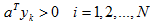在线性不可分的情况下，不等式组不可能同时满足。一种直观的想法就是，希望求一个a*使被错分的样本尽可能少。这种方法通过求解线性不等式组来最小化错分样本数目，通常采用搜索算法求解。

为了避免求解不等式组，通常转化为方程组：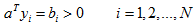矩阵形式为：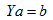。方程组的误差为：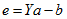，可以求解方程组的最小平方误差求解，即：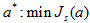Js(a) 即为最小平方误差（Minimum Squared-Error，MSE）的准则函数：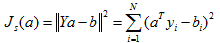准则函数最小化方法

准则函数最小化通常有两种方法：违逆法，梯度下降法。

伪逆法

Js(a) 在极值出对a的梯度为零，即：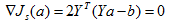于是，得到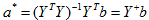，其中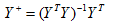是矩阵Y的伪逆。

一个具体的求解示例如下：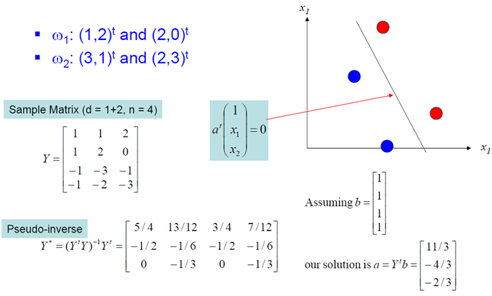梯度下降法

梯度下降法在每次迭代时按照梯度下降方向更新权向量：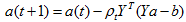直到满足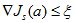或者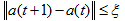时停止迭代，ξ是事先确定的误差灵敏度。

参照感知器算法中的单步修正法，对MSE也可以采用单样本修正法来调整权向量：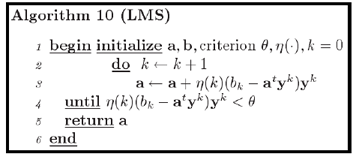这种算法即Widrow-Hoff算法，也称作最小均方根算法或LMS（Least-mean-square algorithm）算法。

（转载请注明作者和出处：http://blog.csdn.net/xiaowei_cqu 未经允许请勿用于商业用途）


展开全文• 误差分析计算公式及matlab代码实现（均方误差MSE，平均绝对误差MAE，平均绝对百分比误差MAPE，均方百分比误差MSPE，均方根误差RMSE，残差平方和SSE）
• 平方误差：表示实验误差大小的偏差平方和。在相同的条件下，各次测定值xi对真实值x的偏差平方后再求和,即： 标准误差（又称为均方根误差RMSE）定义为各测量值误差的平方和的平均值的平方根。 设n个...
• 看了一下机器学习这一节，感觉有点乱，人生观乱了，原来如此。建议本文与贝叶斯一起看。  我们设想一个问题如下：学习器... 最小平方误差用于神经网络权重学习，线性回归以及多项式拟合以及曲线逼近。最大似然估计
• 在这篇博文中，我们主要对比在逻辑斯蒂回归中的**log loss**（对数损失）和**mean squared error**（平均平方误差），并且根据经验和数学分析证明为什么我们推荐使用“log loss”人工智能 逻辑斯蒂回归 损失函数
• 平均绝对偏差（MAE）： 计算公式： 优点：平均绝对误差由于离差被绝对值化，不会出现正负相抵消的情况，因而，平均绝对误差能更好地反映预测值误差的实际情况PCA
•  最小平方误差（又叫最小二乘误差）判别是针对样本线性不可分的情况来讨论的，因此当样本不可分时，就有可能出现错分，就不可能所有样本都满足，对于这种问题，我们有一个约定，就是求解一个解向量使得出现错分的...MSE
• 感知器算法只是当被分模式可用一个特定的判别界面分开时才收敛，在不可分情况下，只要计算程序不终止，它就始终不收敛。 即使在模式可分的情况下，也很难事先算出达到收敛时所需要的迭代次数。...最小平方误差机器学习 数据 函数
• ** 四种方法推导平方公式** 序言: 连续自然数的平方和， Sn=∑k=0nk2=12+22+...+n2S_n = \sum_{k=0}^{n}{k^2} = 1^2 + 2^2 + ... + n^2Sn​=∑k=0n​k2=12+22+...+n2 是我们中学时期便接触到的一个重要公式，当时...
• 残差平方和（SSE） 计算公式： 代码实现： sse = sum((YReal - YPred).^2); 均方误差（MSE） 计算公式： 代码实现： mse = sqrt(sum((YReal - YPred).^2))./2; 平均绝对误差（MAE） 计算公式： 代码实现： mae ...matlab 机器学习
• （1）第一种可以清晰地看到由于正负抵消，总体误差和为0相当于总体没有误差，即使正负不能完全抵消也不符合真实...（3）第三种采用差的平方，使得误差函数平滑连续，并容易计算梯度下降的斜率，越接近最小值梯度越小...神经网络
• 梯度下降法，就是求函数在某一点的导数/偏导数，在该方向上，函数的变化率最大，也就是说，在优化目标函数的时候，只要按照梯度的...基于该公式，我们可以得到误差函数的导数但是在实际的计算过程中，我发现了一个问...
• 简而言之， 使用平方误差更容易求解，但使用绝对误差对离群点更加鲁棒。但是，知其然更要知其所以然！ 每当我们训练机器学习模型时，我们的目标就是找到最小化损失函数的点。当然，当预测值正好等于真实值时，这两个...
• 证明: 总离差平方和=回归平方和+误差平方和。 SST=SSR+SSE,S S T=S S R+S S E,SST=SSR+SSE, 即 ∑i=1n(yi−yˉ)2=∑i=1n(y^i−yˉ)2+∑i=1n(yi−y^i)2 \sum_{i=1}^{n}\left(y_{i}-\bar{y}\right)^{2}=\sum_{i=1}^{n}...大数据
•  这个公式称作最小平方误差（Least Squared Error）。  而确定一条直线，一般只需要确定一个点，并且确定方向即可。   第一步确定点：  假设要在空间中找一点 来代表这n个样本点，“代表”这个词...PCA 数据降维
• 一、曲线拟合问题 给定 N 个输入样本及其标记，对新的样本，给出其标记的预测。 二、曲线拟合的平方和损失函数 ... 平方误差函数是一种广泛使用的误差函数，在曲线拟合问题中，其定义如下： ...最大似然 曲线拟合 高斯噪声
• 最小平方误差理论    假设有这样的二维样本点（红色点），回顾我们前面探讨的是求一条直线，使得样本点投影到直线上的点的方差最大。本质是求直线，那么度量直线求的好不好，不仅仅只有方差最大化的方法。再回想...PCA 主成分分析 最小方差 海量数据 自顶向下
• 我们通过一个预测房价的例子，来给出解决回归问题的一种常用算法函数：平方误差代价函数。（回归问题属于监督学习） 预测房价的例子如下： 已有大量的已知数据（房子大小，房价），将房子大小作为x轴数据，对应的...
• 最近看了BP神经网络(Back Propagation Neural Networks)，对于其中误差反向传播公式的推导比较困惑，在参考周志华老师的《机器学习》和网上一些博客后，做出一个简单的还原。 1. BP网络模型及变量说明 1.1 模型...神经网络 反向传播算法
•  这个公式称作最小平方误差（Least Squared Error）。  而确定一条直线，一般只需要确定一个点，并且确定方向即可。   第一步确定点：  假设要在空间中找一点 来代表这n个样本点，“代表”这个词不是...机器学习
•机器学习
• 3.2 最小平方误差理论    假设有这样的二维样本点（红色点），回顾我们前面探讨的是求一条直线，使得样本点投影到直线上的点的方差最大。本质是求直线，那么度量直线求的好不好，不仅仅只有方差最大化的方法。再...机器学习 计算机视觉 优化 算法 迭代
• 最近看了一下BP神经网络(Backpropagation Neural Networks)，发现很多资料对于BP神经网络的讲解注重原理，而对于反向传播公式的推导介绍的比较简略，故自己根据《PATTERN RECOGNITION AND MACHINE LEARNING》这本书...神经网络 机器学习 模式识别...